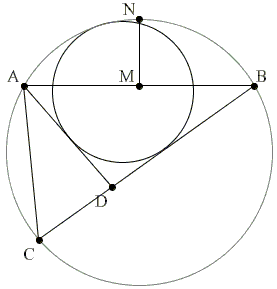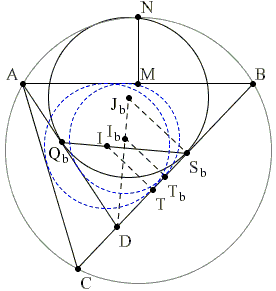## A Hard but Important Sangaku

ABC is a given triangle, and circle O(R) passes through A and B, C lying within the circle. The circle O'(x) lies within O(R), touches AC and BC and also touches O(R) internally. M is the midpoint of AB and N the midpoint of the arc AB, and MN = d.Show that

 x = r + 2d(s - a)(s - b) / cs,

where s is the semiperimeter of ΔABC and r is the radius of the incircle of ΔABC.

The problem is listed as 2.2.8 by Fukagawa and Pedoe and said to be hard but important because of its relevance to a variety of other sangaku. It comes from a third volume of a 1781 collection Seiyo Sanpo by Teisi Fujita (1734-1807).

The solution below is by a New York physicist who posted it under the pseudonym yetti at the MathLinks Forums.

An alternative solution was found by James M. Unger, Professor of East Asian Languages & Literatures, The Ohio State University, Columbus, Ohio.

### References

1. H. Fukagawa, D. Pedoe, Japanese Temple Geometry Problems, The Charles Babbage Research Center, Winnipeg, 1989, pp. 101-102

Write to:

Charles Babbage Research Center
P.O. Box 272, St. Norbert Postal Station
Winnipeg, MBFirst extend, say, BC to cut O(R) in P:This brings the problem into the framework of one of V. Thébault's theorems and suggests a change of notations.Further (re)define a = BC, b = CA, c = AB, d = AD, u = BD, v = DC, where u + v = a. s = (a + b + c)/2 is semiperimeter of ΔABC; sb = (u + c + d)/2 and sc = (v + b + d)/2 the semiperimeters of ΔABD and ΔACD,

 sb + sc = s + d.

The incenter of ΔABC will be denoted I, the incircle (I). For ΔABD, the incenter is Ib, the incircle (Ib) and the inradius rb. We also consider the curvilinear triangles ABD and ACD with the incenters Jb and Jc and incircles (Jb) and (Jc). Note that the unknown x is the radius of (Jb).

In these notations, we want to show that

 (1) x/rb = 1 + 2·MN·(sb - u)(sb - d) / crbsb.

Using Heron's formula for ΔABD, viz.,

 Area( ΔABD)² = sb(sb - u)(sb - d)(sb - c)

we transform (1) into the equivalent

(2)
 x/rb - 1 = 2·MN·(sb - u)(sb - d) / crbsb = 2·MN·(sb - u)(sb - d) / c·Area( ΔABD) = 2·MN·Area( ΔABD) / csb(sb - c) = 2·MN· rb / c(sb - c).

Let now T, Tb, Sb denote the points of tangency of (I), (Ib) and (Jb) with BC and Qb that of (Jb) and AD. By Sawayama's Lemma, I, Sb and Qb are collinear. By definition, DQb = DSb, so that ΔDQbSb is isosceles.If φ = ∠ADB / 2, then ∠ISbD = ∠ISbT = π/2 - φ. Thus in right ΔITSb,

 TSb = IT· tan(φ) = r · tan(φ).

With this in mind,

 DTb = sb - c,

whereas,

 DSb = DT + TSb = DB - TB + TSb = u - (s - b) + r · tan(φ).

Using x = DSbtan(φ) and rb = DTbtan(φ),

 x / rb = DSb / DTb = [u - (s - b) + r · tan(φ)] / (sb - c).

Using

 u - (s - b) = u - (sb + sc - d - b) = (sb - c) - (sc - b)

we see that

 (3) x / rb - 1 = [r · tan(φ) - (sc - b)] / (sb - c).

We have now to show that the right hand side in (3) coincides with the right hand side in (2):

 [r · tan(φ) - (sc - b)] / (sb - c) = 2·MN· rb / c(sb - c)

which is the same as showing that

 (4) r · tan(φ) = 2·MN· rb / c + (sc - b).

Observe that

 ∠MAN = ∠BAN = ∠BCN = ∠ACB / 2

implying

 2·MN / c = MN / (c/2) = tan(∠ACB / 2) = r / (s - c)

from ΔICT. Also, from ΔABD,

 tan(φ) = rb / (sb - c),

the combination of which reduces (4) to the equivalent

 (5) rrb / (sb - c) = rrb / (s - c) + sc - b.

Using (s - c) - (sb - c) = s - sb = sc - d (5) becomes

 (6) rrb(sc - d) / (sb - c)(s - c) = sc - b.

But now again r / (s - c) = tan(∠ACB / 2) = rc / (sc - d) which further upgrades (6) to

 rbrc / (sc - b)(sb - c) = 1

which is the same as

 (7) rb / (sb - c) × rc / (sc - b) = 1.

However, rc / (sc - b) = tan(ψ) where ψ = ∠ADC/2. So that (7) merely asserts that tan(φ)·tan(ψ) = 1 which is obvious in view of φ + ψ = 90°.

Since (7) holds, the equivalent (1) is also true.## Sangaku